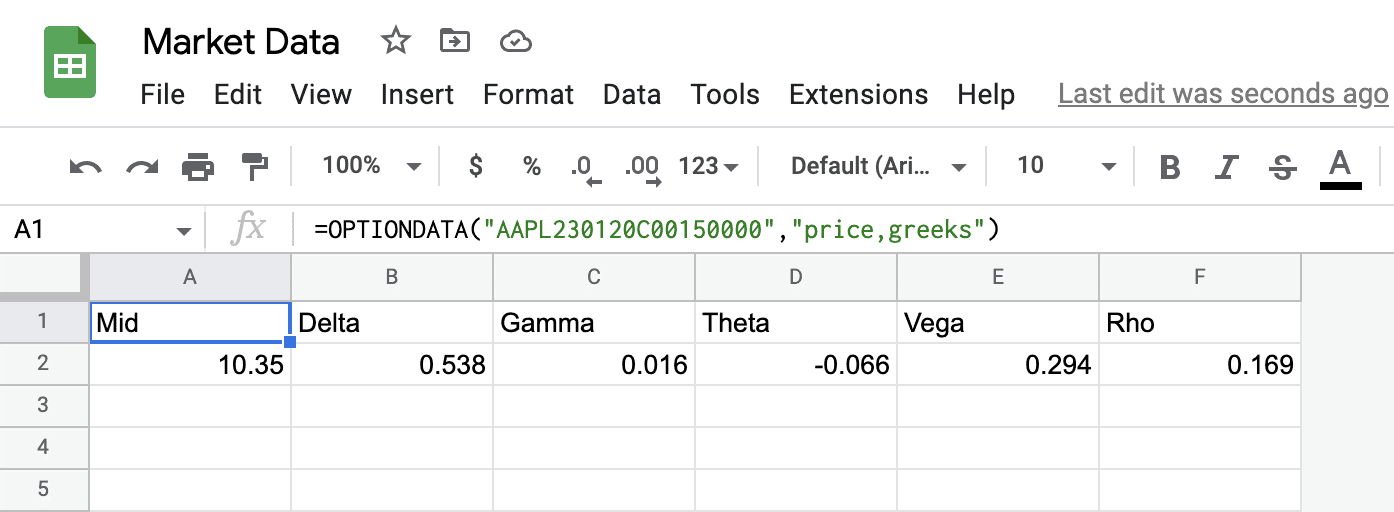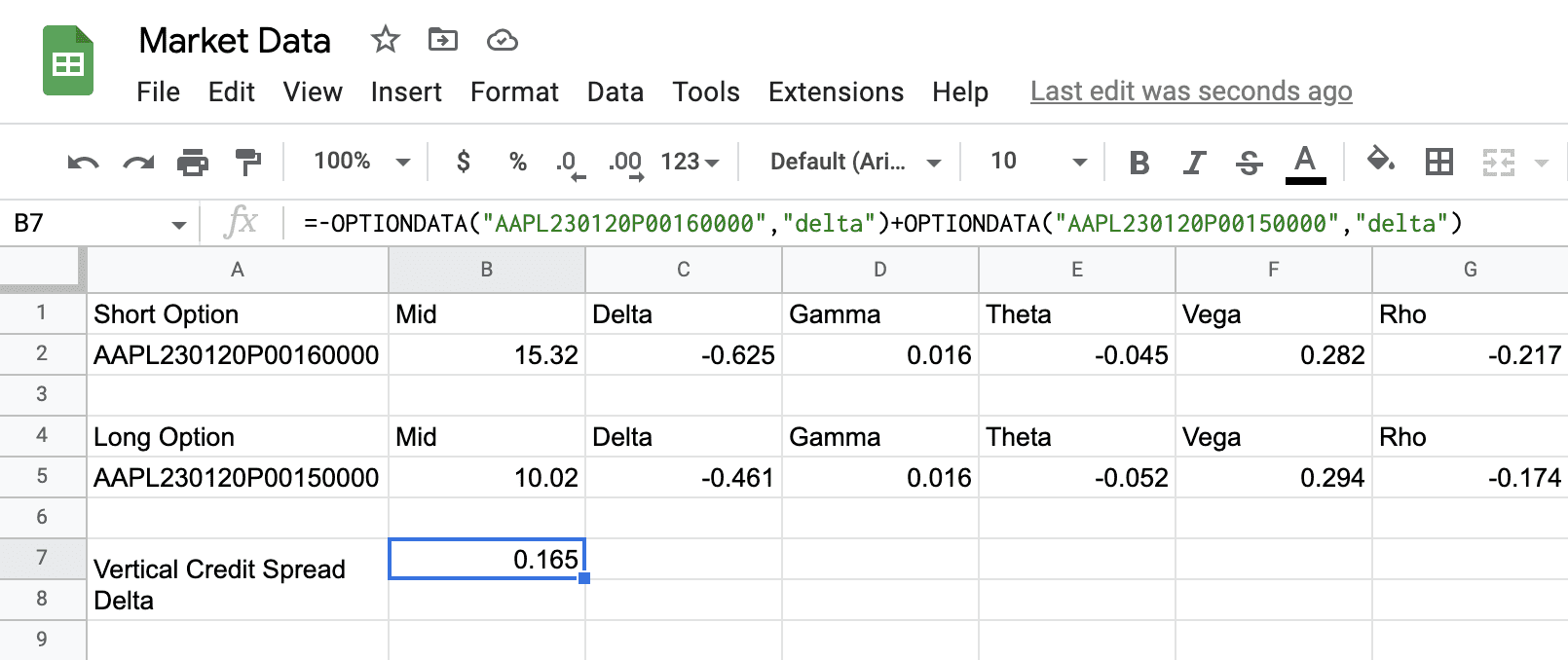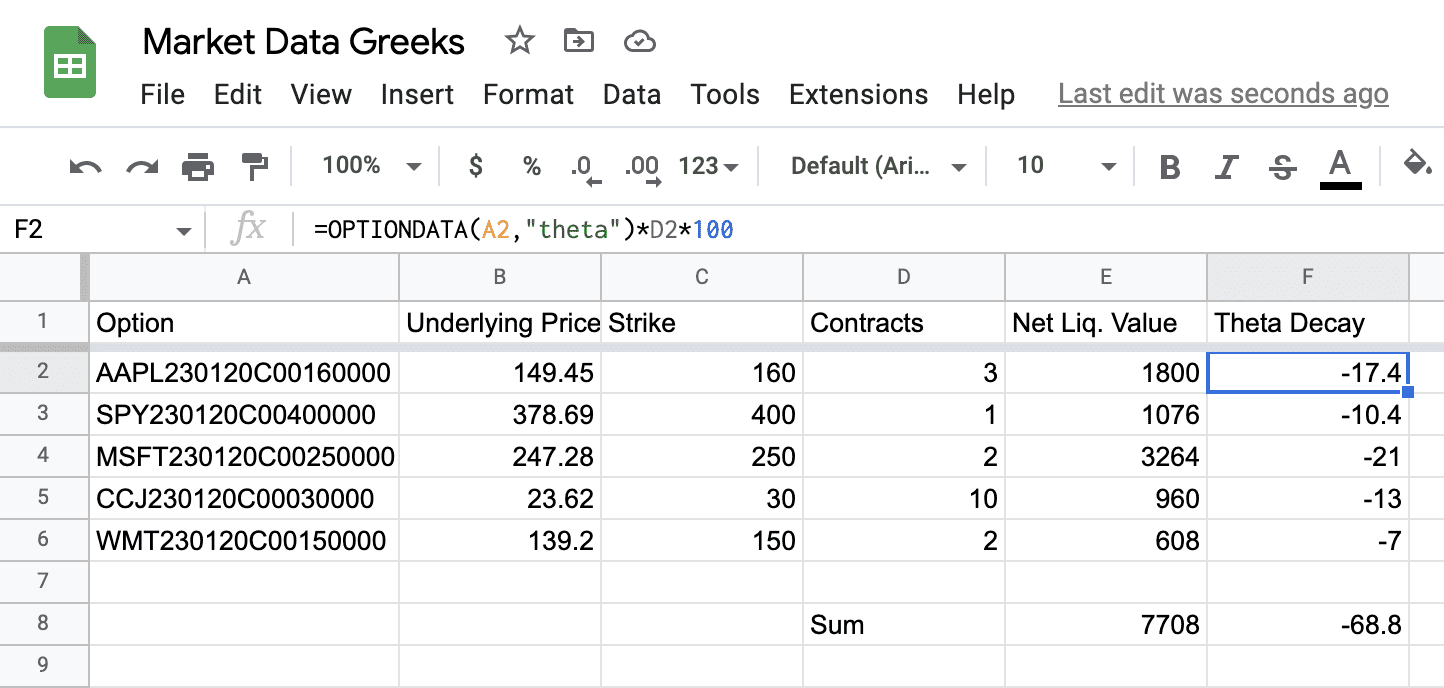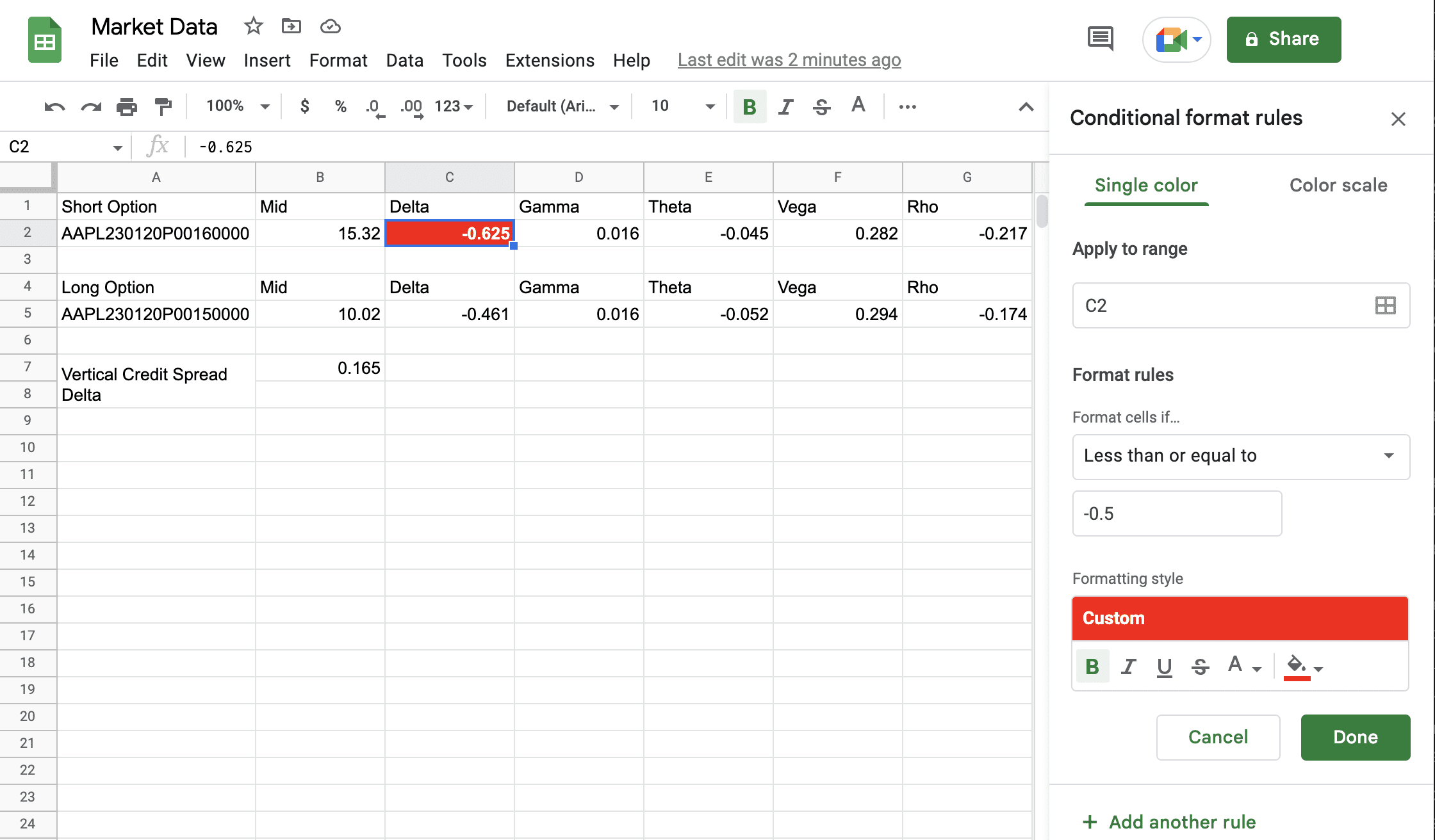The OPTIONDATA formula will calculate the option greeks to your spreadsheet automatically using the keyword "greeks" as a parameter. Risk management has never been easier.

October 24, 2022It is easy to calculate option greeks (Delta, Gamma, Theta, Vega, Rho) in your spreadsheet. Add “greeks” as a parameter to the `OPTIONDATA` formula like this: `=OPTIONDATA("AAPL230120C00150000","price,greeks")`. In addition to the price, this will output the 5 option greeks.

If you don’t need all the greeks, you can request just the ones you need individually: `=OPTIONDATA("AAPL230120C00150000","price,delta,gamma")` would return just delta and gamma along with the price of the option.

### Calculate Option Delta For SpreadsYou can combine multiple OPTIONSDATA formulas to calculate the greeks of your credit spreads, iron condors, and other strategies. Let’s say you are short a \$10 put spread for AAPL at the \$160/150 strikes. You can use two OPTIONDATA formulas to calculate the delta of this spread using the following formula: `=-OPTIONDATA("AAPL230120P00160000","delta") + OPTIONDATA("AAPL230120P00150000","delta")`.

It appears complex, but it is actually surprisingly simple. You just use `-OPTIONDATA("contract","delta")` for the option you are short and `+OPTIONDATA("contract","delta")` for the option your are long. The result is the combined delta of the option spread, which is automatically calculated by combining the delta of the two options.

### Calculating the Daily Loss Due To Theta Decay of A Portfolio of OptionsTheta is the greek that tells us about the time decay of the option. Theta works against option buyers and in favor of option sellers.

If we’re holding a portfolio of calls it can be useful to determine the dollar amount of the theta decay of the portfolio. With Market Data, this is extremely easy to calculate. Just use `=OPTIONDATA("contract symbol", "theta")*quantity of call options*100`. This will show you a dollar amount you are losing each day due to theta decay in your portfolio.

For option writers, just add a negative sign before the `OPTIONDATA` formula (since you are short the option) and you can see how much theta you are collecting per day for writing a portfolio of options. No matter which way you decide to play the theta game, Market Data will keep you informed.Using greeks opens up a whole new realm of possibilities in Google Sheets to easily configure your risk management however you’d like it. Suppose I’d like to be notified when the short end of my option spread gets tested so that I can roll out the spread. Use the Conditional formatting option in the Format menu to easily accomplish this.

In this example, I’ve configured my sheet to highlight in red the delta of the short option when it goes below -0.5. Since that’s happening right now, this cell will be highlighted in my sheet, alerting me that I should be rolling out this credit spread.

Are you worried about getting gamma-squeezed? No problem, just add an alert and don’t let gamma sneak up on you.

Conditional formatting is not a substitute for your broker’s real-time alerts, since they are only displayed if you login to your spreadsheet. However, they can be useful when you are tracking dozens of trades at once since they easily draw your attention to specific contracts or spreads that have exceeded the exact parameters that you’ve set.

Let us know in the comments how you’re using the options greeks in your portfolio.